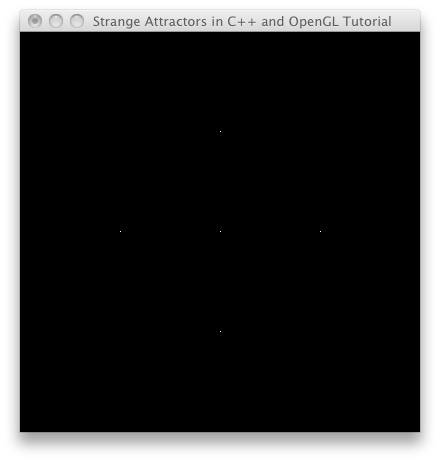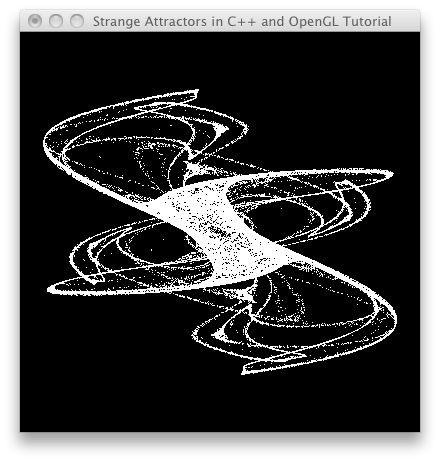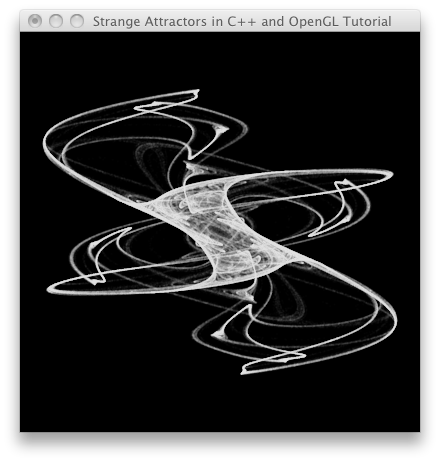# Tutorial: Strange Attractors in C++ and OpenGL

On this page I will show you how to get started rendering strange attractors using C++, OpenGL, and GLUT. The information will be laid out in tutorial form, but you can always skip to the end and get the code. I’m going to assume a basic level of understanding of programming, math, and graphics, focusing on getting you up and running with this specific application of creating chaotic attractors. If I cover something that’s rudimentary to you, just bear with me, and if I skim over something that’s confusing to you, just leave a comment and let me know!

By the way, if you just want to generate strange attractors without programming, there’s a great (and free!) program called Chaoscope that you should take a look at.

## Quick Background

C++: We’ll be using this language because it’s the one I know. But really, we’re just using C functionality in this tutorial, as this introduction to the topic doesn’t justify creating classes or anything else fancy. Global variables and procedural programming will get us generating images more quickly!

OpenGL: We need something besides C++ to show graphics on the screen. OpenGL is great because it’s easy to get (probably already installed), free, and cross-platform (Mac, Windows, Linux, etc).

GLUT: We’ll need a way to initialize a window on the screen to drawing into. I hate dealing with platform specific windowing code, so I use GLUT, which is admittedly old, but is cross-platform and will serve our purposes well for this application.

Strange Attractors: If you’re on this page, I’m assuming you know what a strange attractor is or have at least seen one before. They are fascinating mathematical objects, they are easy to program, and I have used them to create artwork since 2001. I was first introduced to strange attractors in Clifford Pickover’s Chaos in Wonderland. Therefore, we’ll be recreating The King’s Dream, one of the Pickover attractors from this book.

Platform: I’m going to leave out platform specific setup procedures. This means I’m assuming you have OpenGL and GLUT installed and configured to work with your coding environment and know how to at least compile a “Hello World” type console application or command line tool linked to these libraries. I happen to be using XCode on OS X.

Math: When rendering a strange attractor, you are basically visualizing the phase space of a chaotic dynamical system. Many chaotic attractors, such as the Lorenz Attractor, are defined as a set of differential equations. In order to solve and simplify differential equations for programming, you generally have to numerically approximate the system using something like Euler’s method or the Runge-Kutta methods, though we get to skip that step because the Pickover attractor is, in a way, already solved for us. We will be using a set of simple iterated functions that gives us an point for every iteration.

## Step 1: Create a basic OpenGL and GLUT program

It’s always good to start simple. There’s always a bit of headache getting these things going as the linker may complain about undefined symbols, we may forget a header file, etc. So let’s just do the minimal OpenGL and GLUT setup, giving ourselves a keyboard callback function so we can hit ‘Esc’ to exit the program, setting up a 400px x 400px window, and clearing it to black.

``````#include <iostream>
#include <math.h>

#ifdef __APPLE__
#include <opengl>
#include <glut>
#else
#include <gl>
#endif

void myinit() {

// set the background color
glClearColor(0.0f, 0.0f, 0.0f, 1.0f);

// set the foreground (pen) color
glColor4f(1.0f, 1.0f, 1.0f, 1.0f);
}

void mydisplay() {

// clear the screen
glClear(GL_COLOR_BUFFER_BIT);

// swap the buffers
glutSwapBuffers();
}

void mykey(unsigned char mychar, int x, int y) {

// exit the program when the Esc key is pressed
if (mychar == 27) {
exit(0);
}

}

int main (int argc, char **argv) {

// initialize GLUT
glutInit(&argc, argv);

// set up our display mode for color with alpha and double buffering
glutInitDisplayMode(GLUT_RGBA | GLUT_DOUBLE);

// create a 400px x 400px window
glutInitWindowSize(400, 400);
glutCreateWindow("Strange Attractors in C++ and OpenGL Tutorial");

// register our callback functions
glutDisplayFunc(mydisplay);
glutKeyboardFunc(mykey);

// call our initialization function
myinit();

// start the program
glutMainLoop();

return 0;
}
``````

Make sure your code compiles and runs, giving you a black screen 400 x 400 pixels, and exiting when you hit the Esc key.

## Step 2: Create an Appropriate Orthographic Viewport

Now we’ll set up an OpenGL viewport to fill the whole screen, and we’ll manipulate the projection matrix (think of this as camera settings) with the gluOrtho2D function so that the origin (0, 0) is at the center of the screen and the coordinates go from -2.0 to 2.0 in both the x and y axes.

Add the following to the myinit function to set up the viewport and camera:

``````// set up the viewport
glViewport(0, 0, 400, 400);

// set up the projection matrix (the camera)
glMatrixMode(GL_PROJECTION);
gluOrtho2D(-2.0f, 2.0f, -2.0f, 2.0f);

// set up the modelview matrix (the objects)
glMatrixMode(GL_MODELVIEW);
``````

A reality check is always a good idea, so we’ll draw some points – one at the origin, and one in each direction of the graph, 1 unit away from the origin. Add the following to the mydisplay function, after the glClear call:

``````// draw some points
glBegin(GL_POINTS);
glVertex2f(0.0f, 0.0f);
glVertex2f(1.0f, 0.0f);
glVertex2f(0.0f, 1.0f);
glVertex2f(-1.0f, 0.0f);
glVertex2f(0.0f, -1.0f);
glEnd();
``````

Compile and run the code, and you should see something like this:## Step 3: First Pass at the Strange Attractor

Now let’s compute and draw the strange attractor – it’s surprisingly easy. First we’ll need some variables – add these before the myinit function:

``````float	x = 0.1, y = 0.1,		// starting point
a = -0.966918,			// coefficients for "The King's Dream"
b = 2.879879,
c = 0.765145,
d = 0.744728;
int	initialIterations = 100,	// initial number of iterations
// to allow the attractor to settle
iterations = 100000;		// number of times to iterate through
// the functions and draw a point
``````

Next, inside the init function we’ll iterate through the strange attractor equations 100 times to allow the orbit to settle. It takes some iterations for that initial point at (0.1, 0.1) to find its way into the true “phase space” of the attractor. Add this code to the bottom of the myinit function:

``````// compute some initial iterations to settle into the orbit of the attractor
for (int i = 0; i < initialIterations; i++) {

// compute a new point using the strange attractor equations
float xnew = sin(y*b) + c*sin(x*b);
float ynew = sin(x*a) + d*sin(y*a);

// save the new point
x = xnew;
y = ynew;
}
``````

Finally, we’ll actually plot some pixels in the mydisplay function. Replace the glBegin() / glEnd() pair with this:

``````// draw some points
glBegin(GL_POINTS);

// go through the equations many times, drawing a point for each iteration
for (int i = 0; i < iterations; i++) {

// compute a new point using the strange attractor equations
float xnew = sin(y*b) + c*sin(x*b);
float ynew = sin(x*a) + d*sin(y*a);

// save the new point
x = xnew;
y = ynew;

// draw the new point
glVertex2f(x, y);
}

glEnd();
``````

As you can see, the actual strange attractor equations are quite simple. The new point is calculated with two functions, one for x and one for y. Each function contains some trig functions, the variables x and y, and some coefficients (a, b, c, and d, which don’t change). You can think of this like a little machine: insert an x and y value (a point in space), and get a new x and y value. We have to use separate variables for the new x and y since each is dependent on both the previous values of x and y.

Compile and run the code, and you should get something like this:## Step 4: Refining the Image

Congrats! You have rendered a strange attractor. But… it’s not that beautiful. Let’s make some adjustments to improve the quality. First, we’ll change the alpha value of our foreground color, which will allow each pixel to build up the image in layers. We’ll have to turn on blending in OpenGL for this to work. Second, we’ll enable point smoothing in OpenGL to get smoother, antialiased lines. Also, we’ll increase the number of iterations from 100,000 to 1 million.

Set the iterations variable equal to 1000000, and in the myinit function, change or add the following code:

``````// set the foreground (pen) color
glColor4f(1.0f, 1.0f, 1.0f, 0.02f);

// enable blending
glEnable(GL_BLEND);
glBlendFunc(GL_SRC_ALPHA, GL_ONE_MINUS_SRC_ALPHA);

// enable point smoothing
glEnable(GL_POINT_SMOOTH);
glPointSize(1.0f);
``````

Much better! We can see a lot more detail in the mathematical form:## Step 5: Explore!

Here are some ideas to play with once you have this working:

• change the colors
• change the attractor’s coefficients (you may want to choose them randomly to start, although there are some additional calculations you can do to make an educated guess at parameters that create interesting images)
• make some changes to the strange attractor equations (substitute some known equations or make your own)
• modify the code to generate 3d strange attractors
• modify the code to make it interactive or animated
• who says the coefficients have to stay the same for a million iterations? try randomizing or interpolating them.